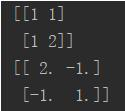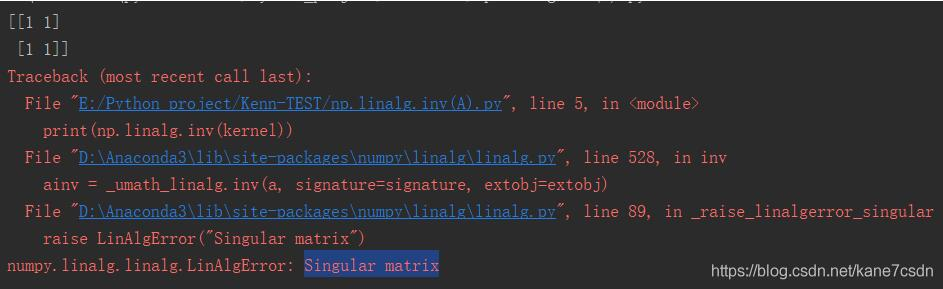• python求解逆矩阵
千次阅读
2021-09-28 18:37:13

方法1：

from numpy import *

import numpy as np

A=np.array([[1,2],[3,4]])
B=np.linalg.inv(A)

print(B)

print(np.dot(B,A))

方法2：



from numpy import *

a1 = mat([[1, 2], [3, 4]])

a2 = a1.I

print(a2)

print(a2*a1)

更多相关内容
• 假定有方阵A， A 的逆矩阵Python 中使用 numpy 库函数命令实现。 import numpy as np A_1 = np.linalg.inv(A) # 逆矩阵（奇异矩阵没有逆矩阵，不可以用此命令计算） A_2 = np.linalg.pinv(A) # 伪逆矩阵...

此问题源于工作中使用多元高斯模型进行异常检测时，计算多元高斯分布 PDF 时涉及到求方阵的逆。

假定有方阵 A，求 A 的逆矩阵。Python 中使用 numpy 库函数命令实现。

import numpy as np

A_1 = np.linalg.inv(A)   # 逆矩阵（奇异矩阵没有逆矩阵，不可以用此命令计算）
A_2 = np.linalg.pinv(A)   # 伪逆矩阵（奇异矩阵也可以使用，但对非奇异矩阵的计算耗时很多）

展开全文开发语言 后端
• Python中，无论是方阵的逆矩阵，还是非方阵的伪逆矩阵，都有现成的模块可供调用

# 前言

在Python中，无论是求方阵的逆矩阵，还是求非方阵的伪逆矩阵，都有现成的模块可供调用。

求方阵的逆矩阵：np.linalg.inv(a)，其中a必须为N×N的方阵，运行结果为a的逆矩阵a-1

求非方阵的伪逆矩阵：scipy.linalg.pinv(a)，其中a可以为任意M×N的矩阵，且M可以不等于N，运行结果为a的伪逆矩阵x，其中x满足：axa=a且xax=x。

# 示例1：求方阵的逆矩阵

from numpy.linalg import inv #求方阵的逆矩阵

a=[[1,2],[3,4]]
inv(a)


输出如下：

array([[-2. ,  1. ],
[ 1.5, -0.5]])


记上述输出为x，则可验证ax=xa=单位矩阵

# 示例2：求非方阵的伪逆矩阵

from scipy.linalg import pinv #求非方阵矩阵的伪逆矩阵

a=[[1,2,3],[4,5,6]]
pinv(a)


输出如下：

array([[-0.94444444,  0.44444444],
[-0.11111111,  0.11111111],
[ 0.72222222, -0.22222222]])


记上述输出为x，则可验证axa=a，xax=x：

import numpy as np

temp1=np.matmul(a,x)
np.matmul(temp1,a)


输出如下：

array([[1., 2., 3.],
[4., 5., 6.]])

import numpy as np

temp1=np.matmul(x,a)
np.matmul(temp1,x)


输出如下：

array([[-0.94444444,  0.44444444],
[-0.11111111,  0.11111111],
[ 0.72222222, -0.22222222]])


END

展开全文• 我就废话不多说了，大家还是直接看代码吧~import numpy as npkernel = np.array([1, 1, 1, 2]).reshape((2, 2))print(kernel)print(np.linalg.inv(kernel))注意，Singular matrix奇异矩阵不可求逆补充：python+numpy...

我就废话不多说了，大家还是直接看代码吧~

import numpy as np

kernel = np.array([1, 1, 1, 2]).reshape((2, 2))

print(kernel)

print(np.linalg.inv(kernel))注意，Singular matrix奇异矩阵不可求逆补充：python+numpy中矩阵的逆和伪逆的区别

定义：

对于矩阵A，如果存在一个矩阵B，使得AB=BA=E，其中E为与A,B同维数的单位阵，就称A为可逆矩阵(或者称A可逆)，并称B是A的逆矩阵，简称逆阵。(此时的逆称为凯利逆)

矩阵A可逆的充分必要条件是|A|≠0。

伪逆矩阵是逆矩阵的广义形式。由于奇异矩阵或非方阵的矩阵不存在逆矩阵，但可以用函数pinv(A)求其伪逆矩阵。

基本语法为X=pinv(A),X=pinv(A,tol),其中tol为误差,pinv为pseudo-inverse的缩写：max(size(A))*norm(A)*eps。

函数返回一个与A的转置矩阵A' 同型的矩阵X，并且满足：AXA=A,XAX=X.此时，称矩阵X为矩阵A的伪逆，也称为广义逆矩阵。

pinv(A)具有inv(A)的部分特性，但不与inv(A)完全等同。

如果A为非奇异方阵，pinv(A)=inv(A)，但却会耗费大量的计算时间，相比较而言，inv(A)花费更少的时间。

代码如下：

1.矩阵求逆

import numpy as np

a = np.array([[1, 2], [3, 4]]) # 初始化一个非奇异矩阵(数组)

print(np.linalg.inv(a)) # 对应于MATLAB中 inv() 函数

# 矩阵对象可以通过 .I 求逆,但必须先使用matirx转化

A = np.matrix(a)

print(A.I)

2.矩阵求伪逆

import numpy as np

# 定义一个奇异阵 A

A = np.zeros((4, 4))

A[0, -1] = 1

A[-1, 0] = -1

A = np.matrix(A)

print(A)

# print(A.I) 将报错，矩阵 A 为奇异矩阵，不可逆

print(np.linalg.pinv(A)) # 求矩阵 A 的伪逆(广义逆矩阵)，对应于MATLAB中 pinv() 函数

这就是矩阵的逆和伪逆的区别

截至2020/10/4，matrix函数还可以使用，但已经过时，应该是mat函数这种。

以上为个人经验，希望能给大家一个参考，也希望大家多多支持我们。如有错误或未考虑完全的地方，望不吝赐教。

时间： 2021-03-09

展开全文• import numpy as np A = np.matrix([[1,2,3],[4,5,6],[7,8,9]]) B = np.array([1,2,3,4,5,6,7,8,9]).reshape((3,3)) print(A) print(B) print("*" * 50) print(np.linalg.inv(A)) print(np.linalg.inv(B)) ...
• ## python求矩阵的逆

千次阅读 2020-03-06 14:51:37
文章目录准备工作 Ready to work环境 Environment模块导入 Module import代数余子式 Algebraic cofactor定义 Definition代码实现 Code余子式 Cofactor代数余子式 Algebraic ...Code矩阵 Inverse of matrix定...numpy 深度学习
• 还在为学习数学而发愁吗？看完这篇文章，希望Python能帮助你消灭数学恐惧症。用NumPy进行线性代数运算线性代数是数学的一个重要分支，比如，我们可以使用线性代数来解决线性回归问题...用NumPy求矩阵在线性代数...
• numpy矩阵运算： import numpy as np A = np.array([1, 2, 3]) T = np.transpose(A) # 矩阵转置 print(A.dot(T)) # 矩阵乘法 B = np.array([[1, 2, 3], [2, 2, 1], [3, 4, 3]]) print(np.linalg.inv(B)) # 矩阵求逆 ...
• A的等于U的乘于L的，L的就利用下三角矩阵求逆算法进行求解，U的可以这样：先将U转置成下三角矩阵，再像对L求逆一样对U的转置求逆，再将得到的结果转置过来，得到的就是U的。因此，关键是下三角矩阵的...
• 矩阵A第i行第j列元素的余矩阵 p=len(A)#矩阵的行数 q=len(A)#矩阵的列数 C=[[A[x][y] for y in ... #按第一行展开递归求矩阵的行列式 p=len(A)#矩阵的行数 q=len(A)#矩阵的列数 if(p==1 and q==1): retur
• 今天小编就为大家分享一篇关于Python最小二乘法矩阵，小编觉得内容挺不错的，现在分享给大家，具有很好的参考价值，需要的朋友一起跟随小编来看看吧
• 主要介绍了python简单实现矩阵的乘，加，转置和运算,结合实例形式分析了Python针对矩阵的乘，加，转置和求逆等运算相关实现技巧,需要的朋友可以参考下
• #模26运算下，求矩阵。 #使用伴随矩阵求矩阵法。 #整个精度保持在整数位（方便求模运算）。 import numpy as np list_A1 = [] #目标矩阵A1 A1 = np.array([[3,13,21,9],[15,10,6,25],[10,17,4,8],[1,23,7,...numpy
• 前提是不使用1numpy等库，自己编写求矩阵，当求一个比较小的矩阵时，结果是正确的，但当矩阵一大（60*60），便出现与用1numpy算出来结果不一样了，不知道是不是精度问题，该如何改进？...
• 正文 现象 首先np.linalg.inv和cv2.invert都是求矩阵，而且要求该矩阵为方阵（行数和列数相同）。 我们先看这个矩阵 import numpy as np import cv2 M1 = np.array([[800.0, 0, 270], [ 0, 820, 223 ],...opencv 图像处理 android
• ## Python中求矩阵的逆

万次阅读 2018-11-26 19:48:25
import numpy as np kernel = np.array([1, 1, 1, 2]).reshape((2, 2)) print(kernel) print(np.linalg.inv(kernel)) 注意，Singular matrix奇异矩阵不可求逆
• 4X4矩阵求逆： import numpy as np A = np.mat("1 2 3 0; 4 5 6 0; 9 8 10 0;0 0 0 1") print("A\n", A) inverse = np.linalg.inv(A) print("inverse: \n", inverse)
• 我在python中做矩阵求逆，结果因数据规模不同而不同，这很奇怪。在在下面的代码中，预计A_inv/B_inv=B/A，但它表明A_inv/B_inv与B/A之间的差异随着数据规模的增大而越来越大。。。这是因为Python不能精确计算大值...
• python中有很多功能强大的模块，这篇经验告诉你，如何使用python的numpy模块创建矩阵，并矩阵逆矩阵。工具/原料windows系统电脑一台python软件并安装numpy模块方法/步骤1第一步，点击键盘 win+r，打开运行窗口...
• python矩阵求逆矩阵相乘，求解，和矩阵转化等有关矩阵算法
• 今天小编就为大家分享一篇Python根据欧拉角旋转矩阵的实例，具有很好的参考价值，希望对大家有所帮助。一起跟随小编过来看看吧
• import sympy from sympy import * t= symbols('t') px1, py1, pz1, vx1, vy1, vz1, ax1, ay1, az1 = symbols('px1 py1 pz1 vx1 vy1 vz1 ax1 ay1 az1') px2, py2, pz2, vx2, vy2, vz2, ax2, ay2, az2 = symbols('px2......